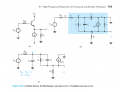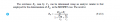# Open circuit time constant example. Cant work it out. Help!

#### kingjamesthethird

Joined Feb 20, 2015
9
Hi

I was wondering if anybody on here has ever seen a text where open and short circuit time constants are FULLY explained. I have been trying to learn the method of obtaining them but they seem to veer from being very easy to very difficult.

i am talking about using them in amplifier analysis for the internal transistor capacitances (e.g Cpi) which come into effect at high frequencies.

I would very much like to see these derived in full and not just stated (often with the patronising pre-text of ''we can clearly see'')

So if anybody knows of a good resource (Not sedra smith) that takes the time to walk you through at least a couple of hard examples I would be very happy.

here is a great example of this. If someone can derive this Rpi properly I would realy appreciate it!#### WBahn

Joined Mar 31, 2012
29,506
I'm not sure exactly what you are asking for, but I think this might help. In analyzing some transistor circuits we use a "dominant pole" assumption (and often design the circuit so that this assumption is valid). This basically means that at the edge of the passband it is a single pole that causes the rolloff. Within the passband, each capacitor falls into one of two camps -- it either looks like an open circuit or it looks like a short circuit. Much of the time you can pretty easily determine which camp each capacitor falls into. If not, you can always search it out by starting at either a low enough frequency that all of the capacitors look like opens or at a high enough frequency that all of them look like shorts. If you start at low frequency then go through capacitor by capacitor and see what the resistance is that it sees when all of the other capacitors look like opens. Whichever one has the biggest time constant will be the capacitor that transitions from open-like to short-like first. You can then treat that capacitor like a short from that point on and continue the process to find the one that will transition next. Keep repeating this until you have identified the order in which all the capacitances will transition from low-frequency behavior to high-frequency behavior.

#### kingjamesthethird

Joined Feb 20, 2015
9
Thanks.
what im asking for is how the resistance seen by Rpi in the above example is found

the example is from sedra smith but there is no explanation for how many of these resistances are found.

would be great if someone could do a step by step of the above example. I think i am missing a fundamental concept regarding transistor terminal resistances or something

#### kingjamesthethird

Joined Feb 20, 2015
9
To find Rpi you need to use this equivalent circuit
View attachment 95134

And Rpi = Vx/Ix
great thanks. thats what i did. at least i used the right approach. i couldnt work out Vrsig or VrL in terms of Vx. hit a wall

#### anhnha

Joined Apr 19, 2012
905
Hi Jony,
I derived Rπ as your last post. The result is not exactly same as the one in Sedra Smith above.
R'L/rπ is omitted in the denominator of Rπ.
It is a bit confusing because R'sig/rπ is remained while R'L/rπ is omitted.
By the way, why Cμ is not considered here?

Last edited:

#### Jony130

Joined Feb 17, 2009
5,475
Hi Jony,
I derived Rπ as your last post. The result is not exactly same as the one in Sedra Smith above.
R'L/rπ is omitted in the denominator of Rπ.
It is a bit confused because R'sig/rπ is remained while R'L/rπ is omitted.
Because there is a error in my last equation.
Vb = Vx - (I1 - Ic) - notice that the result do not have the unit of voltage,
I simply skipped RL in the equation
Vb = Vx - (I1 - Ic)*RL
By the way, why Cμ is not considered here?
Try Google "Open circuit time constant method"

•anhnha

#### anhnha

Joined Apr 19, 2012
905
Jony, I did know that you missed the load resistance and already added in my calculation but the result is different than what is in the book.

#### Jony130

Joined Feb 17, 2009
5,475

#### anhnha

Joined Apr 19, 2012
905
Well, I checked it several times. I am not at home now so I will check it again when I get home.

#### Jony130

Joined Feb 17, 2009
5,475
The mathematica software give me this result

$$\frac{R_L + R_{sig}}{1+gm*R_L}||R_\pi=\frac{R_\pi*(R_L+R_{sig})}{R_L + R_\pi + gm*R_L*R_\pi+R_{sig}}$$

And for Rpi = Rsig = RL = 1KΩ, gm=0.1, β = 100, re = α/gm = 9.90099Ω
And I got exactly the same result as in the book.

•anhnha

#### anhnha

Joined Apr 19, 2012
905
If you compare the formula above with the one in the book, the RL stands alone in denominator of your formula should omitted to get the same result as in the book. That is the reason I am confused.

#### The Electrician

Joined Oct 9, 2007
2,953
Hi

I was wondering if anybody on here has ever seen a text where open and short circuit time constants are FULLY explained.

#### anhnha

Joined Apr 19, 2012
905

#### Jony130

Joined Feb 17, 2009
5,475
Don't forget about me. Or maybe you upload the file here, so that everyone can have a look.

#### The Electrician

Joined Oct 9, 2007
2,953
•anhnha

#### anhnha

Joined Apr 19, 2012
905
Ah, I see where is my mistake now. I used gm = 1/re instead of gm = 1/re - 1/ rpi as indicated by The Electrician.

#### MrAl

Joined Jun 17, 2014
10,904
Hi,

My question is why doesnt the schematic show re when it shows everything else.
I almost made a mistake because of that too